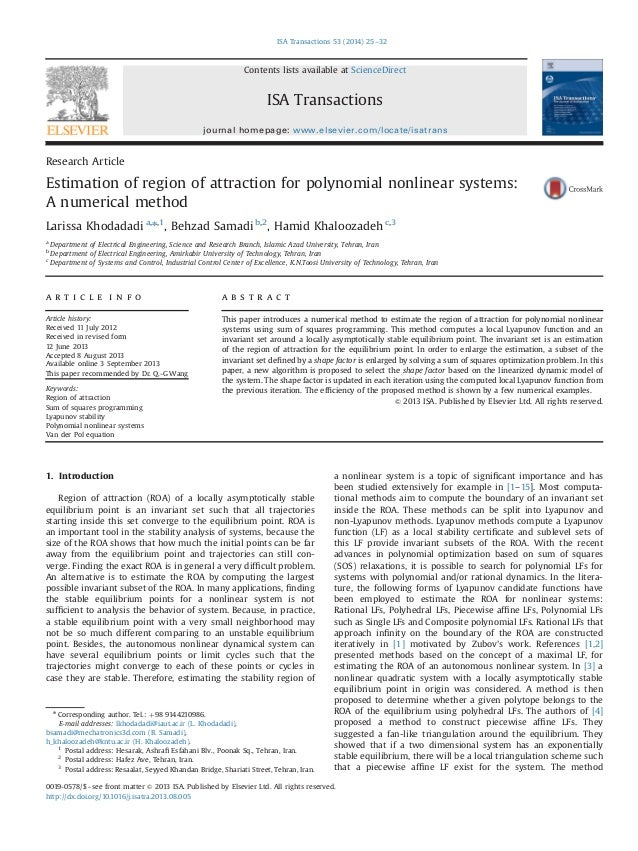Successfully reported this slideshow.Upcoming SlideShare
×

# Estimation of region of attraction for polynomial nonlinear systems a numerical method

488 views

Published on

This paper introduces a numerical method to estimate the region of attraction for polynomial nonlinear systems using sum of squares programming. This method computes a local Lyapunov function and an invariant set around a locally asymptotically stable equilibrium point. The invariant set is an estimation of the region of attraction for the equilibrium point. In order to enlarge the estimation, a subset of the invariant set defined by a shape factor is enlarged by solving a sum of squares optimization problem. In this paper, a new algorithm is proposed to select the shape factor based on the linearized dynamic model of the system. The shape factor is updated in each iteration using the computed local Lyapunov function from the previous iteration. The efficiency of the proposed method is shown by a few numerical examples.

• Full Name
Comment goes here.

Are you sure you want to Yes No• Be the first to comment

• Be the first to like this